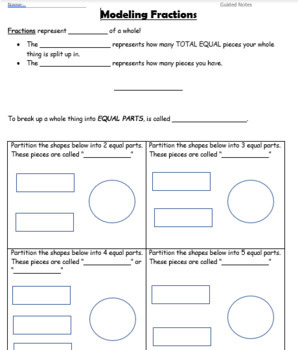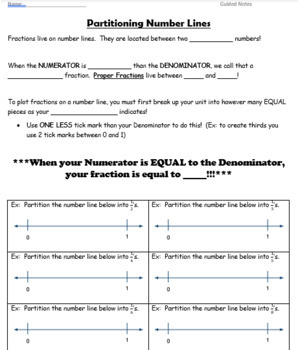# Modeling Fractions GUIDED NOTESSubject
Resource Type
File Type

Zip

(545 KB|2 pages)
Product Rating
Standards
Also included in:
1. Entire Unit of Guided Notes on the fundamentals of fractions. Modeling FractionsEstimating FractionsComparing FractionsEquivalent FractionsEquivalent Fractions Area ModelsEquivalent Fractions Number LinesSimplifying FractionsMixed NumbersImproper FractionsCompare & Order Fractions & Mixed N
\$20.50
\$15.00
Save \$5.50
• Product Description
• StandardsNEW

Guided Notes on Modeling Fractions using Area Models & Number Lines (focus on partitioning number lines). Answer Key included.

Decompose a fraction into a sum of fractions with the same denominator in more than one way, recording each decomposition by an equation. Justify decompositions, e.g., by using a visual fraction model. Examples: 3/8 = 1/8 + 1/8 + 1/8; 3/8 = 1/8 + 2/8; 2 1/8 = 1 + 1 + 1/8 = 8/8 + 8/8 + 1/8.
Understand a fraction 𝘢/𝘣 with 𝘢 > 1 as a sum of fractions 1/𝘣.
Explain why a fraction 𝘢/𝘣 is equivalent to a fraction (𝘯 × 𝘢)/(𝘯 × 𝘣) by using visual fraction models, with attention to how the number and size of the parts differ even though the two fractions themselves are the same size. Use this principle to recognize and generate equivalent fractions.
Represent a fraction 𝘢/𝘣 on a number line diagram by marking off 𝘢 lengths 1/𝘣 from 0. Recognize that the resulting interval has size 𝘢/𝘣 and that its endpoint locates the number 𝘢/𝘣 on the number line.
Represent a fraction 1/𝘣 on a number line diagram by defining the interval from 0 to 1 as the whole and partitioning it into 𝘣 equal parts. Recognize that each part has size 1/𝘣 and that the endpoint of the part based at 0 locates the number 1/𝘣 on the number line.
Total Pages
2 pages
Included
Teaching Duration
N/A
Report this Resource to TpT
Reported resources will be reviewed by our team. Report this resource to let us know if this resource violates TpT’s content guidelines.